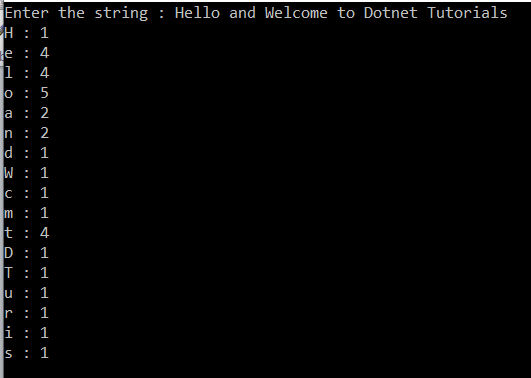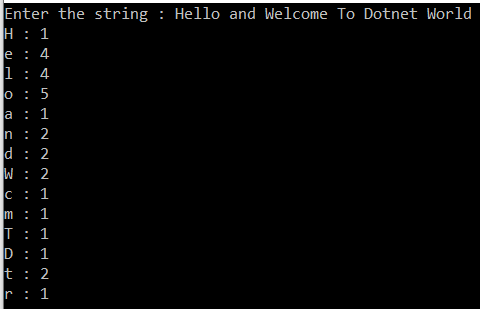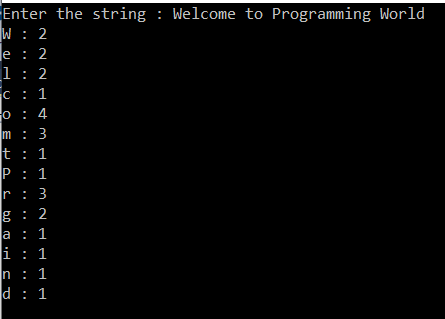# Character Occurrence in a String in C#

## Character Occurrence in a String in C# with Examples

In this article, I am going to discuss the Character Occurrence in a String in C# program with some examples. Please read our previous article where we discussed Binary to Decimal Conversion Program in C# with some examples. This is one of the most frequently asked interview questions in the interview. Here as part of this article, we are going to discuss the following mechanisms to count the character occurrences in a string.

1. Using Loop to Count the Character Occurrence in a String.
2. How to use Dictionary to Count the Number of Occurrences of character in C#.
3. Using LINQ Group By method to count character occurrences.
##### Using Loop to Count the Character Occurrence in a String in C#:

Here in the following program, we take the input from Console and then remove the blank spaces from the input if any. Check the length of the input message and if it is greater than 0, then using for loop we calculate the number of occurrences of each character,

```using System;
namespace LogicalProgram
{
class Program
{
static void Main(string[] args)
{
Console.Write("Enter the string : ");

//Remove the empty spaces from the message
message = message.Replace(" ", string.Empty);

while (message.Length > 0)
{
Console.Write(message + " : ");
int count = 0;
for (int j = 0; j < message.Length; j++)
{
if (message == message[j])
{
count++;
}
}
Console.WriteLine(count);
message = message.Replace(message.ToString(), string.Empty);
}

}
}
}```

Output:##### Using Dictionary to Count the Number of Occurrences of character in C#:

In the below program, we created a dictionary to store each character and its count. We take the input message from the console and then loop through each character of the input message. For each character, we check whether that character already there in the dictionary or not. If already there then just increased its count by 1 else add that character to the dictionary with count as 1.

```using System;
using System.Collections.Generic;

namespace LogicalProgram
{
class Program
{
static void Main(string[] args)
{
Console.Write("Enter the string : ");

Dictionary<char, int> dict = new Dictionary<char, int>();

foreach (char ch in message.Replace(" ", string.Empty))
{
if (dict.ContainsKey(ch))
{
dict[ch] = dict[ch] + 1;
}
else
{
}
}

foreach (var item in dict.Keys)
{
Console.WriteLine(item + " : " + dict[item]);
}

}
}
}```

Output:##### Using LINQ Group By method to count character occurrences in C#:

In the following program, we take the input message from the console and replace the blank spaces with an empty string. Then we use Linq Group By method to group each character and convert the result into a dictionary where character is the key and the number of counts is its value.

```using System;
using System.Collections.Generic;
using System.Linq;

namespace LogicalProgram
{
class Program
{
static void Main(string[] args)
{
Console.Write("Enter the string : ");

Dictionary<char, int> dict = message.Replace(" ", string.Empty)
.GroupBy(c => c)
.ToDictionary(gr => gr.Key, gr => gr.Count());

foreach (var item in dict.Keys)
{
Console.WriteLine(item + " : " + dict[item]);
}